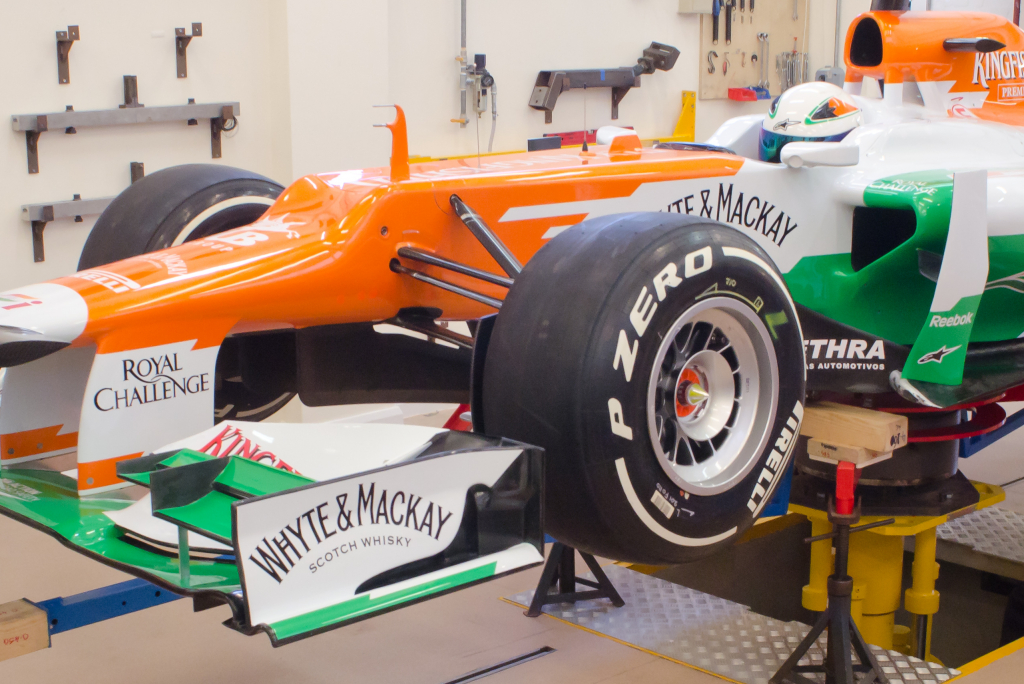This unique facility measures the moment of inertia and centre of gravity.

If an engineer was faced with the issue of obtaining the centre of gravity of a road vehicle, an easy option would be to check a 3D CAD model and an answer would be forthcoming. The centre of gravity would be obtained very quickly, probably referenced to the co-ordinate system of the vehicle. There would also be further data giving the moments of inertia tensor and the principal axes.

However, the figures quoted would have been obtained from the computer model which may have been more suited for crash modelling and the accuracy of certain components may have been compromised. As the moments of inertia of a vehicle are made up of the sum of all the individual parts own local inertia multiplied by the distance squared. Any component located the furthest distance from the centre of gravity needs to be modelled accurately.

#### Specification:

• Mechanical measurement of Inertia and Centre of Gravity
• Tried and tested methodology used for the last 30 years based on inverted pendulum
• Dimension range of objects 6.5 (l) x 2.8 (w)x 2(h)
• Centre of gravity in three axis
• Moments of Inertia in three axis
• Principal Inertias.

#### Typical applications include:

• F1 vehicles